Introduction to Fixed Income Valuation | IFT World
IFT Notes for Level I CFA® Program
IFT Notes for Level I CFA® Program

# Part 4

Before discussing yield measures for money market instruments, it is important to understand the concept of periodicity. Periodicity is the number of compounding periods in a year, or number of coupon payments made in a year.

The stated annual rate for a bond will depend on the periodicity we are assuming. The stated annual rate is also called the annual percentage rate or APR.

Instructor’s Note

A quarterly coupon paying bond has a periodicity of four, while a semi-annual bond has a periodicity of two, and a monthly-pay bond with a given annual yield would have a periodicity of twelve.

“Compounding more frequently within the year results in a lower (more negative) yield-to-maturity.”

Consider a 5-year, zero-coupon bond priced at 80 per 100 par value. What is the stated annual rate for periodicity = 4, periodicity = 2, and periodicity = 1?

When periodicity = 4: compounding happens four times a year. N = 20; (5 years x 4 = 20). PMT = 0 as it is a zero-coupon bond. PV = -80; FV = 100; CPT I/Y = 1.12. This is the rate for each quarter. The stated annual rate is 1.12 x 4 = 4.487%.

When periodicity = 2: N = 10; PV = -80; PMT = 0; FV= 100; CPT I/Y = 2.2565. The stated annual rate is 2.25 x 2 = 4.51%.

When periodicity = 1: N = 5; PV = -80; PMT = 0; FV= 100; CPT I/Y = 4.56%. With a periodicity of 1, the stated annual rate is the same as the effective annual rate.

The formula for conversion based on periodicity is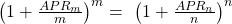Example

A 4-year, 3.75% semi-annual coupon payment government bond is priced at 97.5. Calculate the annual yield to maturity stated on a semi-annual bond basis and convert the annual yield to:

1. An annual rate comparable to bonds that make quarterly coupon payments.
2. An annual rate comparable to bonds that make annual coupon payments.

Solution to 1:

The stated annual yield to maturity on a semiannual bond basis can be calculated using a financial calculator: N = 8; PMT = 1.875; FV = 100; PV = -97.5; CPT I/Y. I/Y = 2.2195%. Hence, the stated annual yield to maturity = 2.2195% x 2 = 4.439%.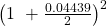=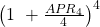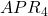= 4.415%

The annual rate of 4.439% for compounding semiannually compares with 4.415% for compounding quarterly.
Solution to 2: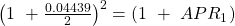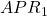= 4.488%

The annual percentage rate of 4.439% for compounding semiannually compares with an effective annual rate of 4.488%.

The effective annual rate (EAR) is the yield on an investment in one year taking into account the effects of compounding. This rate has a periodicity of one as there is only one compounding period per year. EAR is used to compare the rate of return on investments with different frequency of compounding (periodicities).

Semiannual bond equivalent yield: Yield per semi-annual period times two. If the yield per semi-annual period is 2%, then the semi-annual bond equivalent yield is 4%.

### 3.4 Yield Measures for Fixed-Rate Bonds

• Street convention: It is the yield to maturity using a 30/360 day convention assuming payments are made on scheduled dates, even if the payment date fell on a weekend or a holiday.
• True yield: Yield to maturity calculated using an actual calendar of weekends and holidays. For instance, assume the coupon date falls on 15 March 2015, which is a Sunday. Street convention assumes the payment is made on that date, whereas true yield assumes the payment is made on 16 March if it is a business day. The coupon payment is discounted back from 16 March instead of 15 March.
• Government equivalent yield: Yield to maturity calculated using the actual day/count convention used for U.S. Treasuries.
• Current yield: Sum of the coupon payments received over the year divided by the flat price. It is also called the income or interest yield. Example: A 5-year, 8% semiannual coupon payment bond is priced at $960. Its current yield is 80/960 = 0.0833 = 8.33%. Current yield is not an accurate measure of the rate of return as it ignores the frequency of coupon payments, reinvestment income, and capital gain/loss on a bond.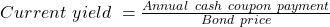• Yield-to-call: Calculates the rate of return on a callable bond if it is bought at market price and held until the call date. The difference between YTM and the yield-to-call is that YTM assumes the bond is held to maturity. Calculation of yield-to-call is the same as YTM where N = number of periods to call date and FV= call price. • Yield-to-first call (YTFC): It is the internal rate of return if the bond was bought at market price and held until the first call date. • Yield-to-second call: Similarly, the yield on a callable bond if it was bought at market price and held to the second call date is called yield-to-second call. • Yield-to-worst: Yield is calculated for every scenario. The lowest yield is called the yield-to-worst. Example An analyst observes the following statistics for two bonds:  Bond A Bond B Annual Coupon Rate 6.00% 10.00% Coupon Payment Frequency Semi-annually Quarterly Years to Maturity 4 years 4 years Price (per 100 par value) 95 110 Current Yield ? ? Yield to Maturity ? ? 1. Calculate both yield measures for the two bonds. 2. How much additional compensation, in terms of yield to maturity, does a buyer of Bond A receive for bearing additional risk compared with Bond B Solution to 1: The current yield for Bond A is 6/95 = 6.316% and the yield to maturity for Bond A is 7.469%. The current yield for Bond B is 10/110 = 9.091% and the yield to maturity for Bond B is 7.106%. Solution to 2: Compare the yields for the same periodicity to answer this question. 7.106% for a periodicity of four converts to 7.169% for a periodicity of two. The additional compensation for the greater risk in Bond A is 30 bps (0.07469 – 0.07169). Example Consider a bond which is selling for$100 and has the following cash flows:

Calculate the yield-to-first call and yield-to-second call.

Solution:

The yield-to-first call can be calculated as follows: PV= -100; N = 5; PMT = 10; FV = 102; CPT I/Y; I/Y = 10.325%. YTFC = 10.325%.

The yield-to-second call in our example will be 10.09%: PV= -100; N = 8; PMT = 10; FV = 101; CPT I/Y; I/Y = 10.09%.

Example

A bond with 4 years remaining until maturity is currently trading for 101.75 per 100 of par value. The bond offers a 5% coupon rate with interest paid semiannually. The bond is first callable in 2 years and is callable after that date on coupon dates according to the following schedule:

 End of Year Call Price 2 102.50 3 101.50 4 100.00
1. What is the bond’s annual yield-to-first-call?
2. What is the bond’s yield-to-worst?

Solution to 1:

The yield-to-first-call can be calculated with the following key strokes:

PV = -101.75, FV = 102.5, N = 4, PMT = 2.5, CPT I/Y = 2.6342.

To arrive at the annualized yield-to-first-call, the semiannual rate must be multiplied by two. (2.6342 × 2 = 5.2684)

Solution to 2:

The yield-to-worst is 4.52%. The bond’s yield to worst is the lowest of the sequence of yields-to-call and the yield to maturity. Yield-to-first-call = 5.27%, Yield-to-second-call = 4.84%, and Yield to maturity = 4.52%.

** IFT Website Un-available on Monday 2-October-2023, 0700-1200 GMT **
This is default text for notification bar